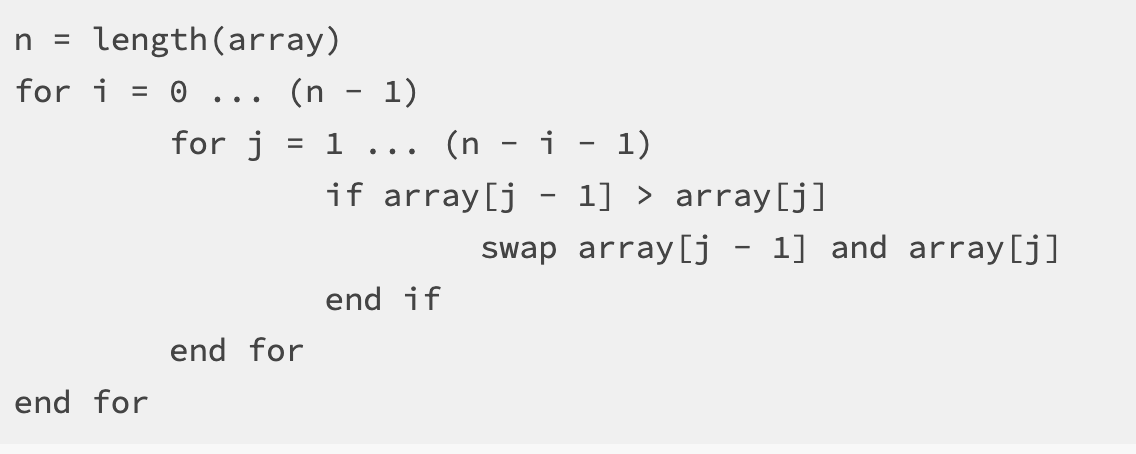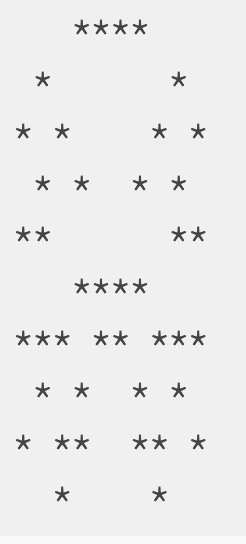# Assignment 3: Array Puzzles¶

## Arrays Bonus Video¶

Still a confused about arrays? Watch this bonus demonstration by former 131 TA Amanda Hua on how to create, write to, read from, and get the length of arrays.

## Assignment Setup¶

To create your repository go here. Then follow the same accept/import process described in the setup instructions.

## Array Puzzles¶

You are given three problems to complete below. For each problem we supply you examples of what the output could look like.

## Bubble Sort¶

Bubble Sort is a simple, well known, algorithm that can be used to sort an array. Implementing a simple sorting algorithm such as bubble sort is often seen as a rite of passage for the novice computer scientist.

The way that bubble sort works is by “bubbling” the larger values up. If a value is larger than its neighbor it will be swapped until all of the larger values make their way to the end of the array and the smaller values end up at the beginning of the array.

Your task is to create a program called `BubbleSort.java` in the `assignment3` package. This program should first use `Scanner` to ask the user how big they would like the array to be. It will then prompt the user to enter that many integer values, which get stored into the array.

You should then sort this array in ascending order using the bubble sort algorithm. To assist you, take a look at this pseudocode implementation of the sorting algorithm:Notice that the above code does not look like Java code that we have seen before. If you were to put this into Eclipse, it would not work. This “pseudocode” captures the essence of the task at hand, which a programmer (in this case, you!) can translate into a more specific computer language like Java or python (or even a human language like Spanish or Chinese….what’s the difference between human language and computer language, anyway?).

For a visualization of how this algorithm works, take a look at this.

You should print out the original array as well as the sorted array so that the results can be easily verified.

Note that the rubric requests that you step through bubble sort in the debugger as part of the demo process. You are highly encouraged to practice this before you demo!

Example Output:

`Given values:  8 6 7 5 3 0 9`

`Sorted values: 0 3 5 6 7 8 9`

## Frequency Table¶

Make a program called `FrequencyTable.java` in the `assignment3` package. This program will generate `amount` number of integers between 1 and `max` where `amount` and `max` are values supplied by the user through `Scanner`.

Your program should create a frequency table, counting the number of times that each number is randomly generated. Think carefully about how you can use an array for this purpose. How big should our array be? Can we define a relationship between the random values that are generated and how we count them in the array?

Some iterative development tips:

• Try making your array before you write the code for generating random numbers. Try using the array to print out information with the same structure as the example below (even though the frequencies will all be 0). Make sure the numbers start at 1 and go up to exactly `max`.

• Try writing code that will generate a random number in your range. Print out the result. If `max` is 1, the generated number should always be 1. If `max` is 2, the generated number should sometimes be 1 and sometimes be 2. You can use these two values as a litmus test to see if your generator is working. (Once you have a number generator working, you can delete the print statement.)

• Try using the number you generated as your index in the array and increase that count by one. Is there an index out of bounds error? Why might that be happening, and how could you fix it?

Example Output:

`Frequencies for 100 randomly generated values between 1 and 10`

`1: 10`

`2: 9`

`3: 10`

`4: 9`

`5: 10`

`6: 6`

`7: 15`

`8: 15`

`9: 8`

`10: 8`

## Symmetrical Image¶

Create a program `SymmetricalImage.java`. This program should create an `n` x `m` array where `n` and `m` are values supplied by the user through `Scanner`.

We wish to use this array to create some randomly generated vertically symmetrical images. To do this, first select a random point within the 2D array. You will then need to compute the “mirrored” point on the other side of the array. Both of these array positions should be marked.

For example, if the size of the array is 10x10 and the point that I have randomly selected is at position (2, 3), then the corresponding “mirrored” point would be at position (2, 6) and both of these positions would be marked in the array. Note that if (2, 6) was the randomly generated point that (2, 3) is still its mirrored point - your code should work in both situations. If you’re having trouble understanding how this works then sketch out a simple example on paper and make sure you understand it before moving on!

You should generate `n * m / 4` random points. It is OK if you randomly select the same point more than once, this will just add to the random flavor of the resulting images.

Finally, you should print out the image by going through the entire array and printing a `*` if the value in the given position is marked and a blank space otherwise.

Example output:

`A randomly generated, symmetrical 10 x 10 image:`